# 基于ALBERT和同义词词林的主观题自动评分方法Automatic Scoring Method for Subjective Questions Based on ALBERT and Cilin

DOI: 10.12677/CSA.2020.109177, PDF, HTML, XML, 下载: 174  浏览: 458  科研立项经费支持

Abstract: Aiming at the machine automatic scoring of subjective questions with reference answers, both the fit of the score points and the overall similarity of the text must be considered. An automatic scoring method for subjective questions that combines ALBERT and Cilin is proposed. First use ALBERT’s Fine-tuning method to calculate the textual semantic similarity between the reference answer and the test taker’s answer. Then use the keyword extraction operation to calculate the score-oriented keyword similarity between the two texts using Cilin. Finally, the comprehensive score is calculated by combining semantic similarity and keyword similarity. Comparative experiments on real data sets show that the method in this paper has a significant improvement in scoring accuracy.

1. 引言

2. ALBERT模型

ALBERT是Google公司AI团队发布的预训练语言模型 ，采用双向的Transformer编码器结构 ，如图1所示。其中：Ii表示单个词或字的向量输入，Trm即Transformer，Ti表示最终隐藏层输出，通过编码器里的注意力矩阵和注意力加权后，每个Ti都具有整句话上下文的语义信息。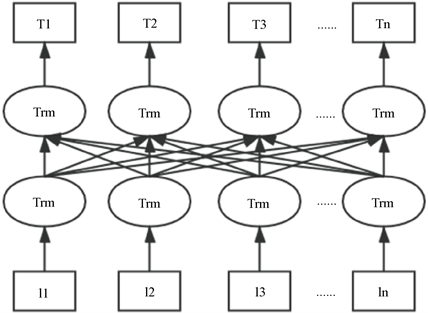Figure 1. ALBERT model structure

Transformer是Encoder-Deocder结构的seq2seq模型，ALBERT模型使用了Transformer的Encoder层提取输入序列的特征信息，Encoder层由Self-Attention层、Feed Forward层和两个Add & Norm层组成，如图2所示。输入向量序列时，Transformer会把输入词的位置信息向量化后与原词向量相加，让输入词带有位置信息。之后编码器对输入的序列经过Self-Attention处理，通过计算词间关系矩阵并以加权计算的方式更新输入序列的词向量。在Self-Attention层和Feed Forward层后面都连着一个Add & Norm层，该层的操作就是将上一层的输出和Add & Norm层输出直接相加，然后再对相加结果进行归一化。Feed Forward层是一个简单结构的全连接前馈网络。

$\text{Attention}\left(Q,K,V\right)=\text{softmax}\left(\frac{Q{K}^{\text{T}}}{\sqrt{{d}_{k}}}\right)V$ (1)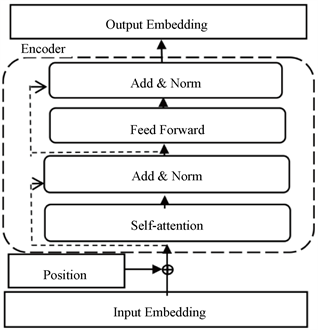Figure 2. Transformer Encoder structure

$\text{MultiHead}\left(Q,K,V\right)=\text{Concat}\left({\text{head}}_{1},\cdots ,{\text{head}}_{h}\right){W}^{o}$ (2)

${\text{head}}_{i}=\text{Attention}\left(Q{W}_{i}^{Q},K{W}_{i}^{K},V{W}_{i}^{V}\right)$ (3)

ALBERT模型使用Transformer的Encoder层学习每个词的上下文信息，获得了更优秀的词向量。除此之外，ALBERT模型还用两个无监督预训练任务强化对词向量的语义表示能力，分别是Masked Language Model (简称MLM)和Sentence-Order Prediction (简称SOP)。

MLM任务：随机选取输入文本中15%的词做三种形式的替换，然后去预测被替换掉的词。形式一是用[Mask]标记替换掉80%的被替换词；形式二是用随机的一个词去代替本来要覆盖的词，随机替换的词占被替换词的10%；形式三是对余下10%的词不进行任何替换。这样设计是为了避免模型在微调时会过度依赖训练数据中不存在的[Mask]标记，同时也让模型在计算词向量的时候更依赖上下文信息。

SOP任务：通过提取同一文本中的两个句子A和B，预测句子中A和B的语序。取正序的A和B为正例，改变A和B的顺序作为反例。因为同一文本中描述的主题相同，所以在同一个文本中选取句子对可以减少文本主题的差异对训练效果的影响。

3. 同义词词林Table 1. Comparison before and after expansion of Cilin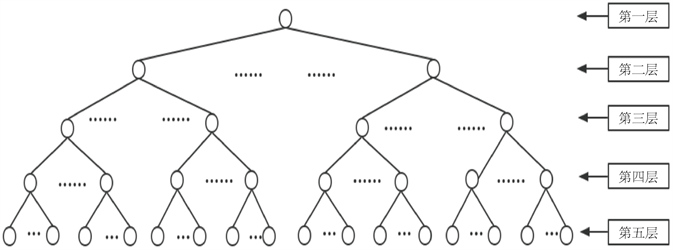Figure 3. The tree-like hierarchical structure of CilinTable 2. Cilin coding rule table

4. 基于ALBERT和同义词词林的主观题自动评分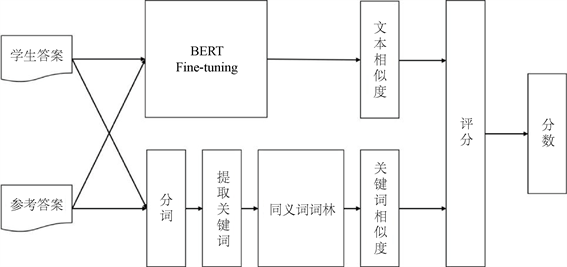Figure 4. Automatic scoring flowchart for subjective questions

4.1. 文本相似度计算

$Z=WX+b$ (4)

${P}_{i}=\frac{{\text{e}}^{{z}_{i}}}{{\sum }_{j=1}^{C}{\text{e}}^{{z}_{j}}}$ (5)

$L=-\left[y\mathrm{log}\stackrel{^}{y}+\left(1-y\right)\mathrm{log}\left(1-\stackrel{^}{y}\right)\right)$ (6)

4.2. 关键词相似度计算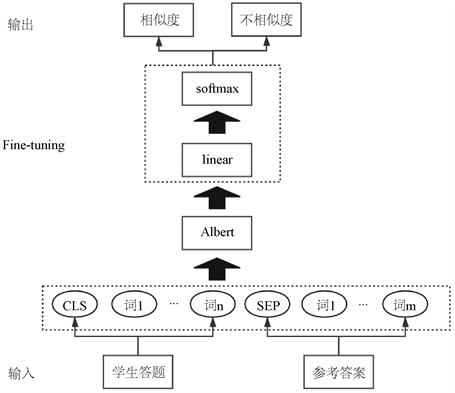Figure 5. Block diagram of text similarity calculation

$sim\left({C}_{1},{C}_{2}\right)=\frac{MaxDIFF-dis\left({C}_{1},{C}_{2}\right)}{MaxDIFF-MinDIFF}$ (7)

$dis\left({C}_{1},{C}_{2}\right)=IC\left({C}_{1}\right)+IC\left({C}_{2}\right)-2IC\left(LCS\left({C}_{1},{C}_{2}\right)\right)$ (8)

$IC\left(C\right)=1-\frac{\mathrm{log}\left(hypo\left(C\right)+1\right)}{\mathrm{log}\left(maxnodes\right)}$ (9)

4.3. 综合评分

$Score=\left\{\begin{array}{l}\left[\alpha ×TextSim+\left(1-\alpha \right)×KeySim\right]×MaxScore,\text{\hspace{0.17em}}\text{\hspace{0.17em}}\text{\hspace{0.17em}}\text{\hspace{0.17em}}\text{\hspace{0.17em}}TextSim\ge \beta \\ KeySim×MaxScore,\text{\hspace{0.17em}}\text{\hspace{0.17em}}\text{\hspace{0.17em}}\text{\hspace{0.17em}}\text{\hspace{0.17em}}\text{\hspace{0.17em}}\text{\hspace{0.17em}}\text{\hspace{0.17em}}\text{\hspace{0.17em}}\text{\hspace{0.17em}}\text{\hspace{0.17em}}\text{\hspace{0.17em}}\text{\hspace{0.17em}}\text{\hspace{0.17em}}\text{\hspace{0.17em}}\text{\hspace{0.17em}}\text{\hspace{0.17em}}\text{\hspace{0.17em}}\text{\hspace{0.17em}}\text{\hspace{0.17em}}\text{\hspace{0.17em}}\text{\hspace{0.17em}}\text{\hspace{0.17em}}\text{\hspace{0.17em}}\text{\hspace{0.17em}}\text{\hspace{0.17em}}\text{\hspace{0.17em}}\text{\hspace{0.17em}}\text{\hspace{0.17em}}\text{\hspace{0.17em}}\text{\hspace{0.17em}}\text{\hspace{0.17em}}\text{\hspace{0.17em}}\text{\hspace{0.17em}}\text{\hspace{0.17em}}\text{\hspace{0.17em}}\text{\hspace{0.17em}}\text{\hspace{0.17em}}TextSim<\beta \end{array}$ (10)

5. 实验

5.1. Fine-Tuning实验

5.2. 自动评分实验

5.2.1. 实验设置

5.2.2. 实验结果分析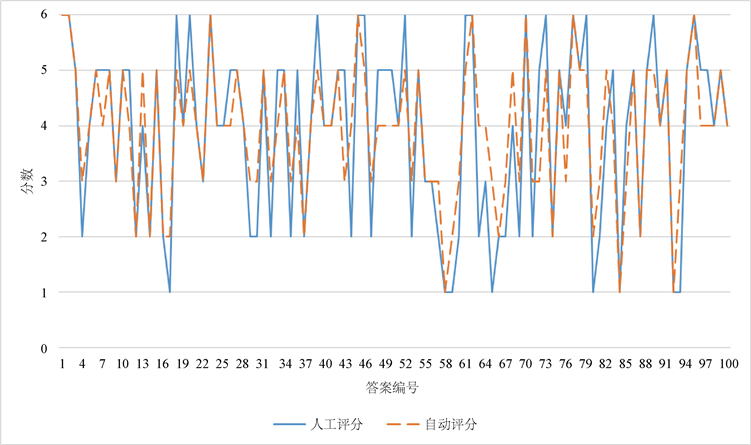Figure 6. Comparison of manual scoring and automatic scoring

6. 总结与展望

NOTES

1https://github.com/brightmart/albert_zh.

2https://ictclas.nlpir.org.

  Page, E.B. (1994) Computer Grading of Student Prose, Using Modern Concepts and Software. The Journal of Experi-mental Education, 62, 127-142. https://doi.org/10.1080/00220973.1994.9943835  高思丹, 袁春风. 语句相似度计算在主观题自动批改技术中的初步应用[J]. 计算机工程与应用, 2004, 40(14): 132-135.  倪应华. 基于XML自动阅卷算法的设计与实现[J]. 仪器仪表学报, 2005, 26(z1): 704-705.  Callear, D.H., Jerrams-Smith, J. and Soh, V. (2001) CAA of Short Non-MCQ Answers. Proceedings of the 5th International Computer Assisted As-sessmentconference, Loughborough, July 2001, 1-14.  王逸凡, 李国平. 基于语义相似度及命名实体识别的主观题自动评分方法[J]. 电子测量技术, 2019, 42(2): 84-87.  罗海蛟, 柯晓华. 基于改进的LDA模型的中文主观题自动评分研究[J]. 计算机科学, 2017, 44(z2): 102-105+128.  周洲, 侯开虎, 姚洪发, 张慧. 基于TF-IDF及LSI模型的主观题自动评分系统研究[J]. 软件, 2019, 40(2): 158-163.  张翠翠, 周国祥, 俞磊, 石雷, 王青青. 基于MVC的试卷生成及主观题判卷算法研究[J]. 系统仿真学报, 2020, 32(1): 105-112.  Devlin, J., Chang, M.W., Lee, K. and Toutanova, K. (2018) Bert: Pre-Training of Deep Bidirectional Transformers for Language Under-standing. arXiv:1810.04805.  Vaswani, A., Shazeer, N., Parmar, N., et al. (2017) Attention Is All You Need. 31st Conference on Neural Information Processing Systems (NIPS 2017), Long Beach, December 2017, 5998-6008.  Lan, Z.Z., Chen, M.D., Goodman, S., Gimpel, K., Sharma, P. and Soricut, R (2019) Albert: A Lite Bert for Self-Supervised Learning of Language Representations. arXiv: 1909.11942.  梅家驹. 同义词词林[M]. 上海: 上海辞书出版社, 1983.  哈工大信息检索研究中心. 同义词词林扩展版[DB/OL]. https://www.ltp-cloud.com/download, 2005.  郭小华, 彭琦, 邓涵, 朱新华. 基于边权重的WordNet词语相似度计算[J]. 计算机工程与应用, 2018, 54(1): 172-178.  彭琦, 朱新华, 陈意山, 孙柳, 李飞. 基于信息内容的词林词语相似度计算[J]. 计算机应用研究, 2018, 35(2): 400-404.  Liu, X, Chen, Q., Deng, C., et al. (2018) LCQMC: A Large-Scale Chinese Question Matching Corpus. Proceedings of the 27th International Conference on Computational Linguistics, Santa Fe, August 2018,1952-1962.